# resonance

(redirected from tympanitic resonance)
Also found in: Dictionary, Thesaurus, Medical, Legal.
Related to tympanitic resonance: tympanic resonance

## resonance,

in acoustics: see vibrationvibration,
in physics, commonly an oscillatory motion—a movement first in one direction and then back again in the opposite direction. It is exhibited, for example, by a swinging pendulum, by the prongs of a tuning fork that has been struck, or by the string of a musical
.

## resonance,

in chemistry: see chemical bondchemical bond,
mechanism whereby atoms combine to form molecules. There is a chemical bond between two atoms or groups of atoms when the forces acting between them are strong enough to lead to the formation of an aggregate with sufficient stability to be regarded as an
.

## Resonance (acoustics and mechanics)

When a mechanical or acoustical system is acted upon by an external periodic driving force whose frequency equals a natural free oscillation frequency of the system, the amplitude of oscillation becomes large and the system is said to be in a state of resonance.

A knowledge of both the resonance frequency and the sharpness of resonance is essential to any discussion of driven vibrating systems. When a vibrating system is sharply resonant, careful tuning is required to obtain the resonance condition. Mechanical standards of frequency must be sharply resonant so that their peak response can easily be determined. In other circumstances, resonance is undesirable. For example, in the faithful recording and reproduction of musical sounds, it is necessary either to have all vibrational resonances of the system outside the band of frequencies being reproduced or to employ heavily damped systems. See Acoustic resonator, Sympathetic vibration, Vibration

## Resonance (quantum mechanics)

An enhanced coupling between quantum states with the same energy. The concept of resonance in quantum mechanics is closely related to resonances in classical physics. See Resonance (acoustics and mechanics)

The matching of frequencies is central to the concept of resonance. An example is provided by waves, acoustic or electromagnetic, of a spectrum of frequencies propagating down a tube or waveguide. If a closed side tube is attached, its characteristic natural frequencies will couple and resonate with waves of those same frequencies propagating down the main tube. This simple illustration provides a description of all resonances, including those in quantum mechanics. The propagation of all quantum entities, whether electrons, nucleons, or other elementary particles, is represented through wave functions and thus is subject to resonant effects. See Acoustic resonator, Harmonic (periodic phenomena)

An important allied element of quantum mechanics lies in its correspondence between frequency and energy. Instead of frequencies, differences between allowed energy levels of a system are considered. In the presence of degeneracy, that is, of different states of the system with the same energy, even the slightest influence results in the system resonating back and forth between the degenerate states. These states may differ in their internal motions or in divisions of the system into subsystems. The above example of wave flow suggests the terminology of channels, each channel being a family of energy levels similar in other respects. These energies are discretely distributed for a closed channel, whereas a continuum of energy levels occurs in open channels whose subsystems can separate to infinity. If all channels are closed, that is, within the realm of bound states, resonance between degenerate states leads to a theme of central importance to quantum chemistry, namely, stabilization by resonance and the resulting formation of resonant bonds. See Degeneracy (quantum mechanics), Energy level (quantum mechanics)

Resonances occur in scattering when at least one channel is closed and one open. Typically, a system is divided into two parts: projectile + target, such as electron + atom or nucleon + nucleus. One channel consists of continuum states with their two parts separated to infinity. The other, closed channel consists of bound states. In the atomic example, a bound state of the full system would be a state of the negative ion and, in the nuclear example, a state of the larger nucleus formed by incorporating one extra nucleon in the target nucleus. See Quantum mechanics, Scattering experiments (atoms and molecules), Scattering experiments (nuclei)

## resonance

A condition prevailing in a system when the frequency of some external stimulus coincides with a natural frequency of the system, and producing a selective or maximum response in the system. Resonance is responsible, for example, for commensurable orbits, the Kirkwood gaps in the asteroid belt, and divisions in Saturn's rings.

## Resonance

(also mesomerism), the nature of the distribution of molecular electron density that may be considered as a partial delocalization of the bonds and atomic charges. Thus, according to the classical structure of the carboxylate anion, one of the oxygen atoms is bonded to the carbon atom by a single bond and carries a full negative charge, whereas the other is connected by a double bond and is neutral. Such a structure (see below) may be given by two equivalent formulas (I) and (II). However, experiment has shown that the oxygen atoms are identical—that is, each atom bears the same partial negative charge, and both bonds to the carbon atom are identical in length. Thus, the actual structure is intermediate between (I) and (II) and may be given as a resonance hybrid of canonical (extreme) structures (I) and (II) or as the resonance structure (III), in which the curved arrows indicate the direction of the electron shifts leading to equalization of the charges and bonds: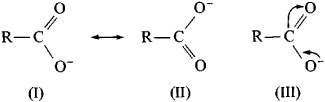Resonance is clearly manifested in conjugate systems. It usually expresses a state that is intermediate between the classical structure and a structure (or structures) with complete charge separation—for example,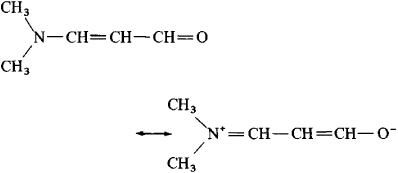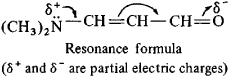In cyclic conjugate systems, a resonance shift does not always lead to charge separation. Thus, the benzene structure may be given as a resonance hybrid of two classical Kekulé structures, (IV) and (V), or as the resonance formula (VI), which reflects the equivalence of the six carbon atoms and bonds between the atoms: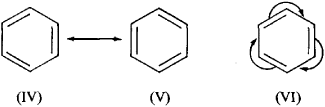The resonance effect is transmitted with only slight loss along a system of conjugate bonds (and therefore is also called the conjugation effect). Chemical substituents with an unshared electron pair (R2N—, RÖ—, HÖ—, and halogens) have a positive resonance effect (+ R effect) and are able to increase the electron density of the rest of the system; groups such as C=O, —C≡N, and —NO2 give a decrease in electron density (— Reffect):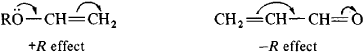The concept of resonance makes possible an explanation of many properties of compounds and reaction mechanisms in organic chemistry. A quantitative picture of the electron density distribution in molecules may be obtained from quantum-mechanical calculations.

The idea of resonance was developed mainly by the English chemist C. Ingold in 1926.

### REFERENCE

Nesmeianov, A. N., and N. A. Nesmeianov. Nachala organicheskoi khimii, book 1. Moscow, 1969.

B. L. DIATKIN

## Resonance

the phenomenon of a marked increase in the amplitude of forced oscillations, occurring in an oscillatory system when the frequency of an external periodic force approaches certain values that are determined by the inherent properties of the system. In the simplest cases, resonance occurs when the frequency of the external force approaches one of the frequencies at which natural oscillations occur as a result of an initial impulse. The nature of the resonance phenomenon is essentially dependent on the properties of the oscillatory system. The simplest resonance occurs in a system subjected to a periodic force when the system’s parameters are not a function of the state of the system itself (a linear system).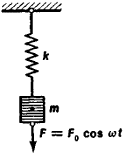Figure 1. Mechanical oscillatory system

The characteristic features of resonance can be seen in the case of a harmonic force acting on a system having one degree of freedom, such as a mass m suspended on a spring and subjected to a harmonic force F = F0 cos ωt (Figure 1) or an electrical network composed of an inductance L connected in series with a capacitance C, a resistance R, and a source of an electromotive force E that varies harmonically (Figure 2). Although the following remarks refer specifically to the first of these models, the explanation can be extended to apply to the second model as well.

We will assume that the spring obeys Hooke’s law (this assumption is necessary if the system is to be linear); that is, the force acting from the spring side on the mass m is equal to kx, where x is the displacement of the mass from its equilibrium position and k is the modulus of elasticity. For the sake of simplicity, the force of gravity is not taken into consideration. Furthermore, assume that when the mass is in motion, it experiences a resistance from the surrounding medium that is proportional to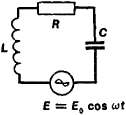Figure 2. Electrical oscillatory system with capacitance C and inductance L connected in series

the velocity ẋ of the mass and to the coefficient of friction b; that is, the resistance is equal to bẋ. This is necessary so that the system will remain linear. Then the equation of motion for the mass m when an external harmonic force F is present has the form

(1) mẍ + bẋ + kx = f0cos wt

where F0 is the amplitude of the oscillations, ω is the angular frequency, equal to 2 π /T, T is the period of the external force, and is the acceleration of the mass m.

The solution of the equation can be represented as the sum of two solutions. The first solution corresponds to the natural oscillations of the system that occur as a result of the action of an initial impulse, and the second solution corresponds to the forced vibrations. As a result of the presence of friction and the resistance of the medium, the natural oscillations are always damped; therefore, over a sufficiently long period of time (the less the damping of the natural oscillations, the longer the time) only certain forced oscillations will continue in the system. The solution corresponding to the forced oscillations has the form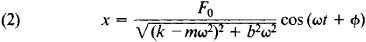Here,Thus, the forced oscillations are harmonic oscillations having a frequency equal to that of the external force. The amplitude and phase of the forced oscillations depend on the relationship between the frequency of the external force and the system’s parameters.

The dependence of the displacement amplitude of the forced oscillations on the relationship between the values of the mass m and the elasticity k can best be seen if one assumes that m and k remain constant while the frequency of the external force is varied. When the frequency is very low (ω → 0), the displacement amplitude x0F0/k. As the frequency ω is increased, the amplitude x0 increases because the denominator in equation (2) becomes smaller. When ω approaches the value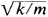(that is, the value of the natural oscillation frequency with low damping), the amplitude of the forced oscillations reaches the maximum, and resonance occurs. With a further increase in ω, the amplitude of the oscillations decreases monotonically, and when ω —> ∞, the amplitude approaches zero.

The amplitude of the oscillations at resonance can be determined approximately by assuming that. Then, x0 = F0/b ω; that is, the lower the damping b in the system, the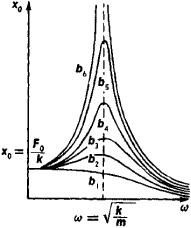Figure 3. Displacement amplitudes as a function of the frequency of an external force for various values of b (b6 < b5 < … < b1)

greater the amplitude of the oscillations at resonance (Figure 3). On the other hand, when the damping in the system is increased, the resonance becomes less and less marked, and if b is very large, then the resonance is no longer noticeable. In terms of energy, resonance is accounted for by the phase relationships between the external force and the forced oscillations that deliver the most power to the system, because the velocity of the system is found to be in phase with the external force and thus creates the most favorable conditions to stimulate forced oscillations.

If a periodic but nonharmonic external force acts on a linear system, then resonance occurs only when the external force contains harmonic components with a frequency close to a natural frequency of the system. In this case, the phenomenon discussed above will proceed for each individual component. If there are several of these harmonic components with frequencies close to a natural frequency of the system, then each of them will produce resonance phenomena, and, in accordance with the principle of superposition, the total effect will be equal to the sum of the effects from the individual harmonic components. If, however, there are no harmonic components in the external force at frequencies close to a natural frequency of the system, then no resonance will occur. Thus, a linear system proves to be resonant only when the external force is harmonic.Figure 4. Electrical oscillatory system with capacitance and inductance connected in parallel

In electrical oscillatory systems composed of a capacitance C connected in series with an inductance L (Figure 2) resonance occurs as follows: when the frequency of the external electromotive force (emf) approaches a natural frequency of the oscillatory system, the amplitudes of the emf across the coil and the voltage on the capacitor taken separately are much greater than the amplitude of the emf created by the source; but the emf across the coil and the voltage on the capacitor are equal to each other in magnitude and opposite in phase. When a harmonic emf acts on a network having a capacitance connected in parallel with an inductance (Figure 4), a special case of resonance occurs—an antiresonance. As the frequency of the external emf approaches the natural frequency of the LC circuit, the amplitude of the forced oscillations in the circuit does not increase; rather, the amplitude of the current in the external net-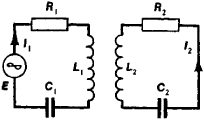Figure 5. Example of two coupled electrical circuits

work feeding the circuit is sharply reduced. In electrical engineering, this phenomenon is called current resonance or parallel resonance. It is attributable to the fact that when the frequency of an external force is close to the natural frequency of the circuit, the reactances of both parallel branches (capacitive and inductive) become identical in magnitude so that the currents in both branches are of approximately the same amplitude but almost opposite in phase. As a result, the amplitude of the current in the external network, which is the algebraic sum of the currents in the individual branches, proves to be much lower than the current amplitudes in the individual branches, which reach their highest values with parallel resonance. As is the case with a series resonance, a parallel resonance increases with a decrease in the active resistance in the branches of the resonant circuit. Series and parallel resonances are known as voltage and current resonances, respectively.

In a linear system having two degrees of freedom, particularly in the case of two coupled systems (for example, in the two coupled electrical circuits in Figure 5), the phenomenon of resonance retains the basic features indicated above. However, because the natural oscillations in a system having two degrees of freedom may occur at two different frequencies (the normal frequencies), resonance occurs when the frequency of a harmonic external force coincides with either one of the system’s normal frequencies. Therefore, if the system’s normal frequencies are not very close to one another, two maxima will be observed in the amplitude of the forced oscillations (Figure 6) as the frequency of the external force is continuously varied. However, if the system’s normal frequencies are close to each other and the system’s damping is sufficiently high so that the resonance at each of the normal frequencies is attenuated, the two maxima may coincide. In this case, the resonance curve for a system having two degrees of freedom loses its “double-humped” character and differs little in appearance from the resonance curve of a linear circuit having one degree of freedom. Thus, the shape of the resonance curve for a system with two degrees of freedom not only depends on the circuit’s damping, as is the case of a system with one degree of freedom, but also on the degree of coupling between the circuits.Figure 6. Resonance curve with two maxima

Coupled systems also exhibit a phenomenon that is to a certain extent analogous to the phenomenon of antiresonance in a system with one degree of freedom. In the case of two coupled oscillatory circuits having different natural frequencies, if the secondary circuit L2C2 is tuned to the frequency of an external emf connected to the primary circuit L1C1 (Figure 5), then the current magnitude in the primary circuit will decrease sharply; the lower the damping in the circuits, the sharper the decrease. The reason for this phenomenon is as follows; when the secondary circuit is tuned to the frequency of the external emf, only enough current appears in the circuit to induce an emf in the primary circuit approximately equal to the external emf in amplitude and opposite in phase.

In linear systems having many degrees of freedom and in continuous systems, resonance retains the same basic features as in a system having two degrees of freedom. However, unlike systems having one degree of freedom, in this case the distribution of the external force with respect to the individual coordinates plays an important role. It is possible to have special instances of distribution of the external force in which resonance does not occur, even though the frequency of an external force coincides with one of the normal frequencies of the system. In terms of energy, this can be explained by certain phase relationships established between the external force and the forced oscillations, such that the power entering the system from the exciting source in one coordinate is equal to the power delivered by the system to the source in another coordinate. An example of this is the excitation of forced oscillations in a string when the external force, which coincides in frequency with one of the normal frequencies of the string, is applied at a point corresponding to a velocity node for the particular normal oscillation (for example, a force that matches the fundamental tone of the string is applied to the very end of the string). Under these conditions, because the external force is applied to a fixed point of the string, the force does not perform work, power does not enter the system from the source of the external force, and there is no appreciable excitation of string oscillations; that is, no resonance is seen.

In oscillatory systems, whose parameters are functions of the state of the system, resonance has a more complicated character in nonlinear systems than in linear systems. The resonance curves in nonlinear systems may be markedly nonsymmetrical, and the phenomenon of resonance can occur for various relationships between the exciting frequencies and the frequencies of small natural oscillations in the system at fractional, multiple, and combined frequencies. Examples of resonance in nonlinear systems are the resonance in ferroresonant circuits, which contain an inductance with a ferromagnetic core, and ferromagnetic resonance, which is associated with the resonance of the elementary (atomic) magnets of a substance when a high-frequency magnetic field is applied.

If an external force causes a periodic change in the energy-storage parameters of an oscillatory system (for instance, the capacitance in an electric circuit), then for certain relationships between the frequencies of the parameter variation and the natural frequency of free oscillations in the system it is possible to have a parametric excitation of oscillations (parametric resonance).

Resonance occurs very frequently in nature, and it plays an important role in technology. Most structures and machines are capable of exhibiting natural oscillations so that a periodic external force may induce resonance. For example, a bridge may resonate as a result of the action of periodic impulses caused by a train passing over rail joints, or the foundation of a structure or a machine itself may resonate because of imperfectly balanced rotating parts in the machine. There have been instances of entire ships becoming resonant at certain speeds of the propeller shafts. In all cases, the resonance causes a marked increase in the amplitude of the forced oscillations throughout the structure and may even result in structural failure. This is an undesirable aspect of resonance; in order to avoid it, the system’s characteristics are selected in such a way that the normal frequencies will be far removed from the possible frequencies of external forces, or else the phenomenon of antiresonance is used in one form or another, for example, by installing dampers or stabilizers. In other cases, resonance plays a positive role; for example, in radio engineering, resonance is almost the only method by which the signals of one radio station (the desired signals) can be separated from signals of all other (interfering) stations.

### REFERENCES

Strelkov, S. P. Vvedenie ν leoriiu kolebanii, 2nd ed. Moscow, 1964.
Gorelik, G. S. Kolebaniia i volny: Vvedenie ν akusliku, radiofiziku i optiku, 2nd ed. Moscow, 1959.

## resonance

[′rez·ən·əns]
(electricity)
A phenomenon exhibited by an alternating-current circuit in which there are relatively large currents near certain frequencies, and a relatively unimpeded oscillation of energy from a potential to a kinetic form; a special case of the physics definition.
(physics)
A phenomenon exhibited by a physical system acted upon by an external periodic driving force, in which the resulting amplitude of oscillation of the system becomes large when the frequency of the driving force approaches a natural free oscillation frequency of the system.
In general, any phenomenon which is greatly enhanced at frequencies or energies that are at or very close to a given characteristic value.
(physical chemistry)
A feature of the valence-bond method that accounts for the anomalies in certain molecules by representing their structures with approximate resonance hybrid formulas; no single electronic formula conforms both to the observed properties and to the octet rule. Also known as mesomerism.
(quantum mechanics)
An enhanced coupling between quantum states with the same energy.
(nucleonics)
(quantum mechanics)

## resonance

The energetic vibration of a body produced by application of a periodic force of nearly the same frequency as that of the free vibration of the affected body. It is the condition of two bodies adjusted to have the same frequency of vibration. There are two types of resonance: sympathetic and ground. Sympathetic resonance is a harmonic beat that develops when the natural vibration frequency of one mechanism is in phase with another mechanism's vibrational frequency. Ground resonance is a self-excited vibration that develops when the landing gear of a rotorcraft repeatedly strikes the ground or deck of a ship, thus unseating the center of mass of the main rotor system. The pounding effect of the landing gear is prone to occur during start-up, ground taxi, rolling takeoff and landing, engine shutdown, and operation from the deck of a rolling or pitching ship. When a helicopter begins to bounce from one wheel to the other in rapid succession, a pendular oscillation of the fuselage sets in. The succession of shocks is transmitted to the main rotor system, causing the main rotor blades to change their angular relationship with each other. This unbalances the main rotor system, which in turn transmits the shock back to the landing gear. This condition, if allowed to continue, can lead to the complete destruction of the helicopter. See also ground resonance.

## resonance

1. sound produced by a body vibrating in sympathy with a neighbouring source of sound
2. the condition of a body or system when it is subjected to a periodic disturbance of the same frequency as the natural frequency of the body or system. At this frequency the system displays an enhanced oscillation or vibration
3. Electronics the condition of an electrical circuit when the frequency is such that the capacitive and inductive reactances are equal in magnitude. In a series circuit there is then maximum alternating current whilst in a parallel circuit there is minimum alternating current
4. Med the sound heard when percussing a hollow bodily structure, esp the chest or abdomen. Change in the quality of the sound often indicates an underlying disease or disorder
5. Chem the phenomenon in which the electronic structure of a molecule can be represented by two or more hypothetical structures involving single, double, and triple chemical bonds. The true structure is considered to be an average of these theoretical structures
6. Physics
a. the condition of a system in which there is a sharp maximum probability for the absorption of electromagnetic radiation or capture of particles
b. a type of elementary particle of extremely short lifetime. Resonances are regarded as excited states of more stable particles
c. a highly transient atomic state formed during a collision process
Site: Follow: Share:
Open / Close